#Function Repository Resource:

# ChiSquareCI

Estimate the confidence interval based on a Chi-squared distribution

Contributed by: Wolfram Research
 ResourceFunction["ChiSquareCI"][var,df] gives a confidence interval based on a χ2 distribution with df degrees of freedom.

## Details and Options

ResourceFunction["ChiSquareCI"][var,df] gives a confidence interval {min,max} for a population variance estimated by var.
For confidence level α,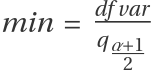and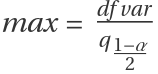, where qi is the ith quantile of a χ2 distribution with df degrees of freedom.
ResourceFunction["ChiSquareCI"] is the confidence interval for a population variance based on a sample variance var estimated from a sample of size df+1.
The following option can be given:
 ConfidenceLevel 0.95 probability associated with a confidence interval

## Examples

### Basic Examples

A 95% confidence interval based on a χ2 distribution:

 In:=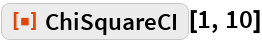Out=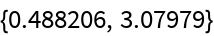### Options

#### ConfidenceLevel

A 99% confidence interval:

 In:=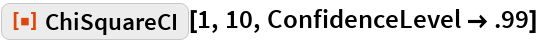Out=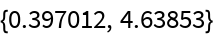## Requirements

Wolfram Language 11.3 (March 2018) or above